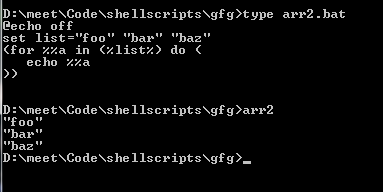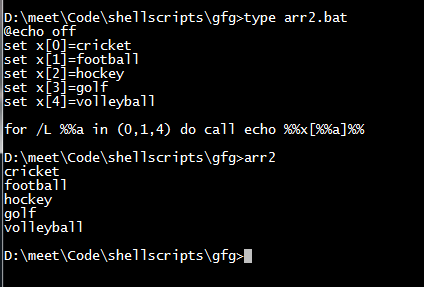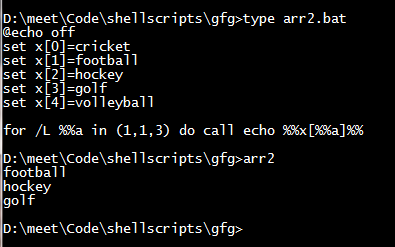GeeksforGeeks App
Open AppBrowser
Continue

# Batch Script – Iterating Over an Array

A batch script is a set of instructions or a script for SOS and Windows Operating System. We can write them in the CMD line by line or we can create a file with a  “.bat” or “.cmd” extension. The file can contain valid instructions for executing by the interpreter. The meaning of batch is the non-interactive execution of the instructions. We can add logic and conditional blocks of programming in such scripts.

## Iterating over Arrays

Iterating over arrays needs to be done in order to write less and maintainable code scripts. We need to iterate the elements of the array one by one or in a particular pattern in such a way that we don’t have to manually echo the elements. We can achieve this in the following ways.

### Method 1:

```@echo off
set list="foo" "bar" "baz"
(for %%a in (%list%) do (
echo %%a
))```In this approach we are using range-based for loops, i.e. we will iterate over the array until it is empty hence the word, for .. in … So after the for loop syntax we can say the keyword, do to execute the commands until the loop is iterable. The contents of the array at every index are stored in the variable “a”, which can be anything as sensible.

Now using this approach we can iterate over arrays using the iterator and then we can echo them. We can modify the contents as well by accessing the original array and index of the element.

## Method 2:

```@echo off
set x=cricket
set x=football
set x=hockey
set x=golf
set x=volleyball

for /L %%a in (0,1,4) do call echo %%x[%%a]%%```The above creates an array of size 5 but it can be any length, the iteration is done in a single line command but is a multi-step process. First, the /L in the for loop will allow iterating through the array elements. The range (0,1,4) is the begin, increment, and end indices for the list.  That is we start with the index 0 and increment 1 after each loop until we hit the number 4 in the index of the list.

After that, we have an inline loop body, which calls the commands to echo and we print the value of x[a] where x is the array variable and a is the iterator in the loop, we enclose the variable in “%%” to get the value of the actual variable which is parsed in. We also use “%%” before the iterator so as to parse in the value of the iterator itself and the enclosed “%%” around the variable to get the value of let’s say “list“.

Let’s take an example of iterating through some elements of the array:

```@echo off
set x=cricket
set x=football
set x=hockey
set x=golf
set x=volleyball

for /L %%a in (1,1,3) do call echo %%x[%%a]%%```Here, we print not all the elements from the array. We use the range as (1,1,3) which iterates from index 1 till 3 one by one. Hence we can achieve multiple combinations with the iterations of the array.

My Personal Notes arrow_drop_up